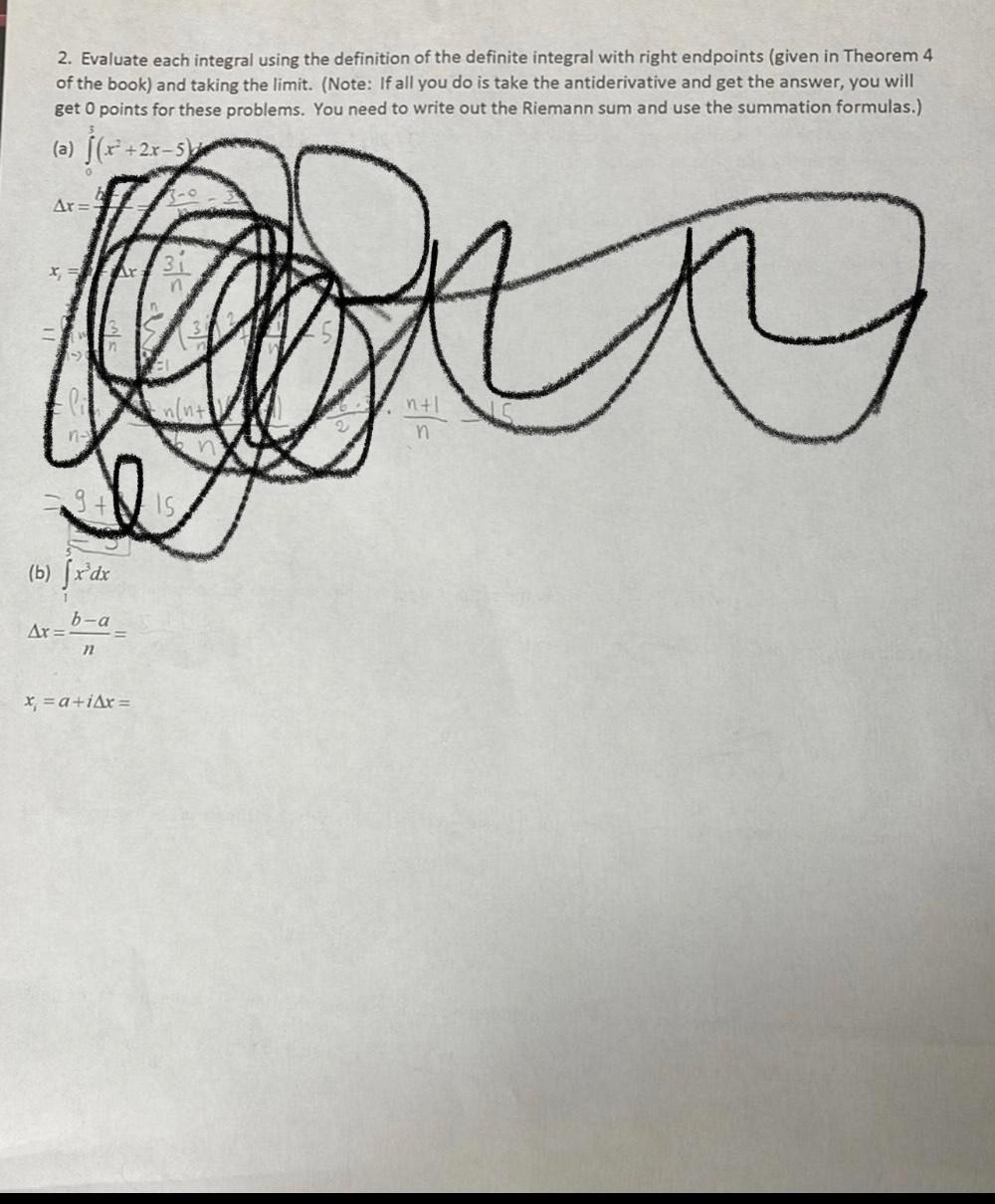Question:

# Evaluate each integral using the definition of the definite integral with right endpoints (given in Theorem 4 of the book) and taking the limit. (a) ∫₀³ (x² +2x-5)dx (b) ∫₁⁵ x³ dxEvaluate each integral using the definition of the definite integral with right endpoints (given in Theorem 4 of the book) and taking the limit. (a) ∫₀³ (x² +2x-5)dx (b) ∫₁⁵ x³ dx